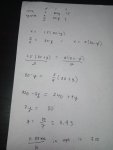# 2 × 2 Linear System Word Problem

#### onesun0000

##### New member
2 × 2 Linear System Word Problem

need help with this 2 × 2 Linear System Word Problem. this is my nephew's homework and he asked my help. I don't know if my solution makes sense or if it's even correct.

Niki owns a small plane that has a speed if 80 km/h without any wind. in 90 minutes, Niki can fly from Virginia to her mansion on a secret island with the wind's help. coming back against the wind, it takes Niki 1 hour to cover half that distance. Find the rate of the wind in mph.

Here's my work. your help would be appreciated.#### Otis

##### Senior Member
Hi. Looks pretty good. You properly added the wind's speed to the plane's speed, on the first half of the round trip (i.e., the wind pushes the plane, increasing the plane's speed), and you subtracted the wind's speed (i.e., flying against the wind decreases the plane's speed), on the return trip.

One comment about notation. Your work shows: x/2 … = x …

That's only true when x = 0, and x cannot equal zero in this exercise. I understand what you were trying to express, but we don't use an equals sign to indicate that one equation follows from another. Use an arrow, instead:

x/2 = 80 - y $$\displaystyle \quad$$ → $$\displaystyle \quad$$ x = 2(80 - y)

Or, simply write your work vertically, and people will understand that the second equation comes from the first (without needing a symbol):

x/2 = 80 - y
x = 2(80 - y)

This exercise seems to be presented for practice working with a system of two equations with two variables (assuming the '2x2 System' title was provided to your nephew). Yet, the problem may be solved with a single variable and equation.

As it took 1 hour to fly half the return distance, the total return trip took 2 hours.

Let w = wind speed

(80 + w)(1.5) = (80 - w)(2)

w ≈ 11.43

Last edited: